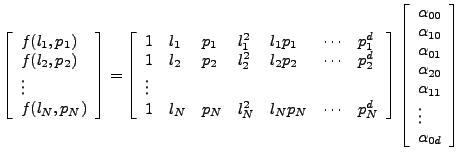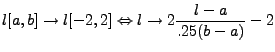Next: Evaluation of polynomials Up: Polynomials Previous: Polynomials   Contents

## Computation of coefficients

Suppose we have 2d data f(l,p), l[1,25000] p[1,5000] and we want to estimate a 2d polynomial D.26 with these data. The system of equations looks like(D29)

The convention used in the Doris software is that we first normalize the data to avoid numerical instabilities (see source utilities.[hc]). The maximum coordinates are that of the original master (stored in the result file of the master image, typically 25000 lines and 5000 range pixels). The coordinates are rescaled to the interval [-2,2].(D30)

(Another way, perhaps a better one (?), would be to make the data zeromean, unit standard deviation) The estimated coefficients thus correspond to the normalized data. For evaluation, the data has to be normalized by the same factors a,b. Normally the information from the master.originalwindow.linelo etc. are used, e.g., for the coregistration and the reference surface polynomial. These numbers can be found in the master result file after the step readfiles at place number of lines original of datafile. A function normalize is called to do the normalization, so it is easy to change the implementation to a different normalization. It has been noticed that for higher order polynomials the normalization factor is very important to obtain a stable estimate.Next: Evaluation of polynomials Up: Polynomials Previous: Polynomials   Contents
Leijen 2009-04-14